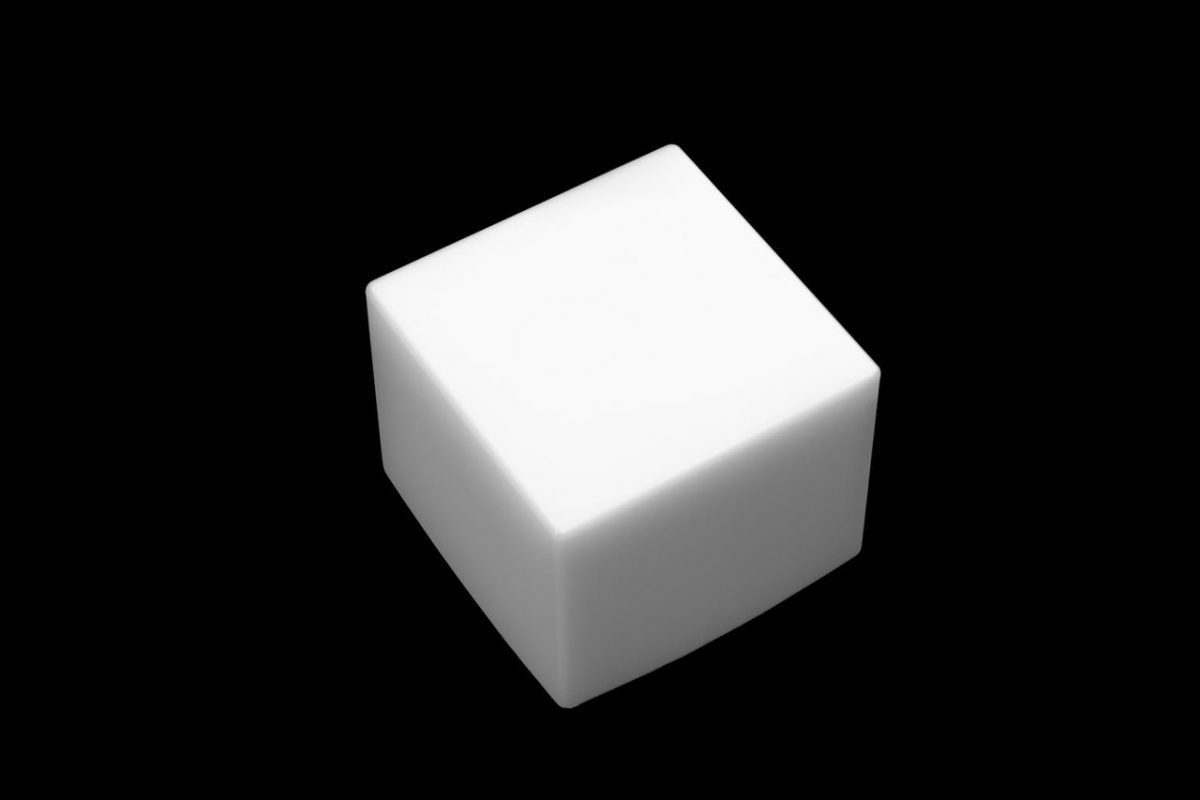# How to Estimate a Normalized Histogram for a 3D ImageBelow I will show you how to estimate a normalized histogram for a 3D image.

In image processing, a histogram shows the number of pixels (or voxels in the case of a 3D image) for each intensity value in a given image.

Let us suppose we have a 3D image that is 512 x 512 x 512 (width x height x depth). This image has 134,217,728 voxels (i.e. 5123).

If we assume the image is grayscale with 256 distinct intensity levels i (where i = 0 [black], 1, 2, …. 253, 254, 255 [white]), the probability p that a voxel chosen at random will have an intensity level i is as follows:

Note that there will be a different probability p for each intensity value of i. For this reason, the i on pi is subscripted.

Let h(i) represent the normalized histogram where h is the count and i is the intensity value. Let K represent the total number of possible intensity values (e.g. 256). The general equation for the normalized histogram is as follows:

Here is the pseudocode for estimating a normalized histogram of a given 3D image of size 5123 with 256 intensity levels:

```// Create the initial unnormalized histogram.
// Initialize all values to 0
for (i = 0; i < 256; i++) {
h(i) = 0;
}

// Traverse each voxel in the image and keep
// a count of the number of times an intensity
// value i appeared.
// w means width, h means height, and d means depth.
for (w = 0; w < 512; w++) {
for (h = 0; h < 512; h++) {
for (d = 0; d < 512; d++) {
// Extract the intensity value for the voxel
i = image[w][h][d];

// Update the histogram for the intensity
// value found above
h(i) = h(i) + 1;
}
}
}

// Now that we have the unnormalized histogram, we have
// to normalize it by dividing each value of the
// histogram by the total number of pixels in the image.
for (i = 0; i < 256; i++) {
h(i) = h(i) / (5123)
}
```

The sum of all the components in the normalized histogram above is equal to 1.